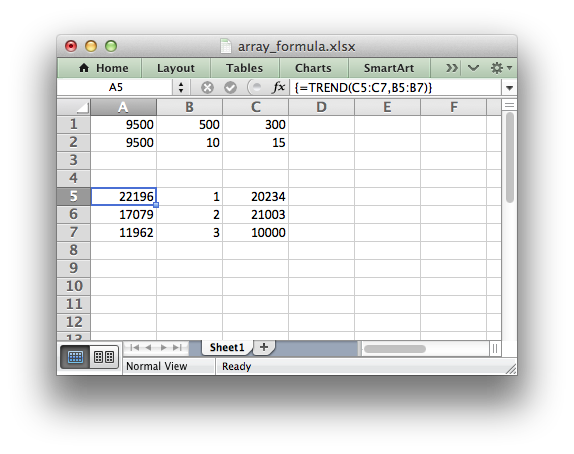libxlsxwriter
array_formula.c

Example of writing array formulas to a worksheet./*
* Example of how to use the libxlsxwriter library to write simple
* array formulas.
*
* Copyright 2014-2021, John McNamara, jmcnamara@cpan.org
*
*/
#include "xlsxwriter.h"
int main() {
/* Create a new workbook and add a worksheet. */
lxw_workbook *workbook = workbook_new("array_formula.xlsx");
/* Write some data for the formulas. */
worksheet_write_number(worksheet, 0, 1, 500, NULL);
worksheet_write_number(worksheet, 1, 1, 10, NULL);
worksheet_write_number(worksheet, 4, 1, 1, NULL);
worksheet_write_number(worksheet, 5, 1, 2, NULL);
worksheet_write_number(worksheet, 6, 1, 3, NULL);
worksheet_write_number(worksheet, 0, 2, 300, NULL);
worksheet_write_number(worksheet, 1, 2, 15, NULL);
worksheet_write_number(worksheet, 4, 2, 20234, NULL);
worksheet_write_number(worksheet, 5, 2, 21003, NULL);
worksheet_write_number(worksheet, 6, 2, 10000, NULL);
/* Write an array formula that returns a single value. */
worksheet_write_array_formula(worksheet, 0, 0, 0, 0, "{=SUM(B1:C1*B2:C2)}", NULL);
/* Similar to above but using the RANGE macro. */
worksheet_write_array_formula(worksheet, RANGE("A2:A2"), "{=SUM(B1:C1*B2:C2)}", NULL);
/* Write an array formula that returns a range of values. */
worksheet_write_array_formula(worksheet, 4, 0, 6, 0, "{=TREND(C5:C7,B5:B7)}", NULL);
workbook_close(workbook);
return 0;
}
workbook_close
lxw_error workbook_close(lxw_workbook *workbook)
Close the Workbook object and write the XLSX file.
worksheet_write_array_formula
lxw_error worksheet_write_array_formula(lxw_worksheet *worksheet, lxw_row_t first_row, lxw_col_t first_col, lxw_row_t last_row, lxw_col_t last_col, const char *formula, lxw_format *format)
Write an array formula to a worksheet cell.
workbook_new
lxw_workbook * workbook_new(const char *filename)
Create a new workbook object.
lxw_worksheet
Struct to represent an Excel worksheet.
Definition: worksheet.h:2107
RANGE
#define RANGE(range)
Convert an Excel A1:B2 range into a (first_row, first_col, last_row, last_col) sequence.
Definition: utility.h:82
lxw_workbook
Struct to represent an Excel workbook.
Definition: workbook.h:292
worksheet_write_number
lxw_error worksheet_write_number(lxw_worksheet *worksheet, lxw_row_t row, lxw_col_t col, double number, lxw_format *format)
Write a number to a worksheet cell.